# 1. Let x, a € R. Prove that if a <a, then -a < x <a. related homework questions

• #### What David Ricardo saw was thát it could still be mutually beneficial for both countries to...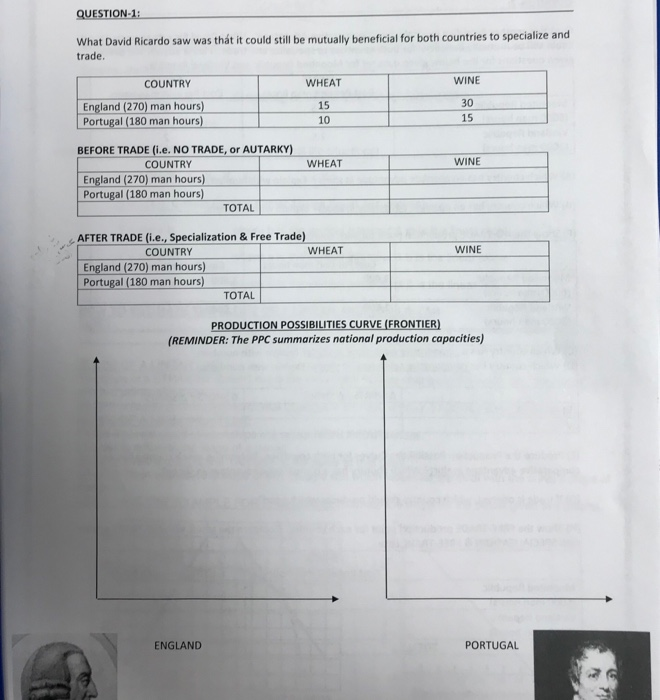What David Ricardo saw was thát it could still be mutually beneficial for both countries to specialize and trade COUNTRY WHEAT WINE England (270) man hours) Portugal (180 man hours 15 10 30 15 BEFORE TRADE (i.e. NO TRADE, or AUTARKY) COUNTRY WHEAT WINE England (270) man hours) Portugal (180 man hours) TOTAL COUNTRY WHEAT WINE England (270) man hours)...

• #### 11. (a) Let F be a field. Prove FixF Rİr (b) Let R be a commutative ring with identity. Prove that one can have R. 11. (a) Let F be a field. Prove FixF Rİr (b) Let R be a commutative ring wi...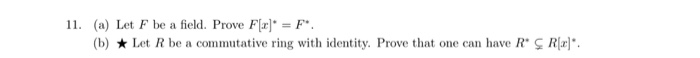11. (a) Let F be a field. Prove FixF Rİr (b) Let R be a commutative ring with identity. Prove that one can have R. 11. (a) Let F be a field. Prove FixF Rİr (b) Let R be a commutative ring with identity. Prove that one can have R.

• #### What number multiplied by 3, then increased by 3/4 of the product, then divided by 7, then diminished by 1/3 of the result, then multiplied by itself, then diminished by 52, whose square root is then extracted before 8 is added, and then divided by 10, gi

What number multiplied by 3, then increased by 3/4 of the product, then divided by 7, then diminished by 1/3 of the result, then multiplied by itself, then diminished by 52, whose square root is then extracted before 8 is added, and then divided by 10, gives the final result of 2?

• #### Let A be an 3 x 4 matrix, and B an 4 x 3 matrix. Prove: If AB Is, then the columns of B are linearly independent. Let A be an 3 x 4 matrix, and B an 4 x 3 matrix. Prove: If AB Is, then the c...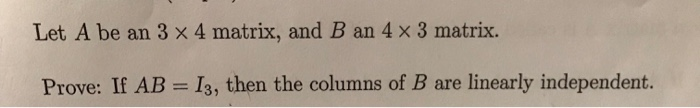Let A be an 3 x 4 matrix, and B an 4 x 3 matrix. Prove: If AB Is, then the columns of B are linearly independent. Let A be an 3 x 4 matrix, and B an 4 x 3 matrix. Prove: If AB Is, then the columns of B are linearly independent.

• #### Additional problem 1 Let AABC be a triangle, let be the bisector of the angle ZCAB Let P be the intersection of and BC. Let R be the point on the line AB such that AR-AC, and let X-APnRC. Let Q denot...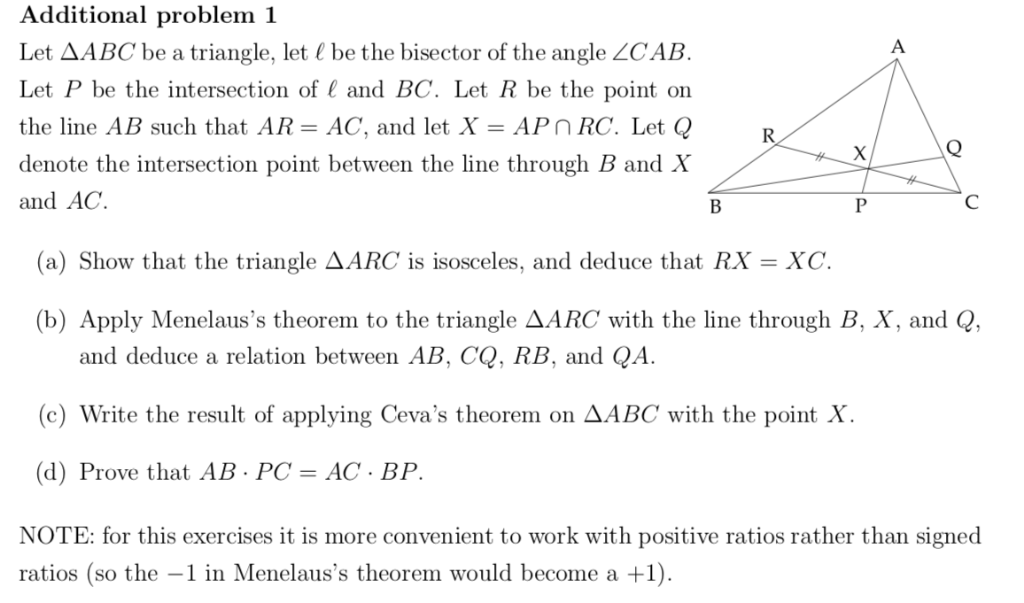Additional problem 1 Let AABC be a triangle, let be the bisector of the angle ZCAB Let P be the intersection of and BC. Let R be the point on the line AB such that AR-AC, and let X-APnRC. Let Q denote the intersection point between the line through B and X and AC. (a) Show that the triangle AARC...

• #### Problem 1. Let A be an m x m matrix. (a) Prove by induction that if A is invertible, then for every n N, An is invertible. (b) Prove that if there exists n N such that An is invertible, then A is inv...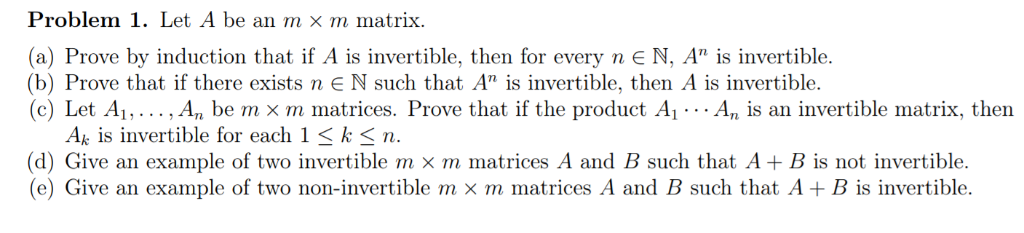Problem 1. Let A be an m x m matrix. (a) Prove by induction that if A is invertible, then for every n N, An is invertible. (b) Prove that if there exists n N such that An is invertible, then A is invertible. (c) Let Ai, . . . , An be m x m matrices. Prove that if...

• #### Number Theory 13 and 14 please! 13)) Let n E N, and let ā, x, y E Zn. Prove that if ā + x = ā + y, then x-y. 14. In this exercise, you will prove that the additive inverse of any element of Z, is uni...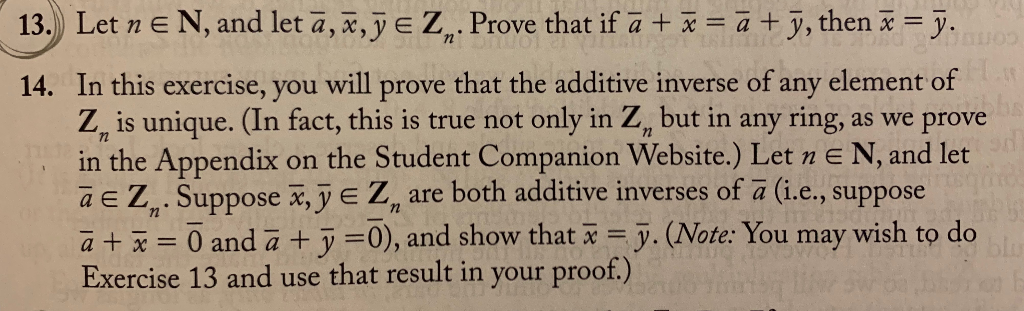Number Theory 13 and 14 please! 13)) Let n E N, and let ā, x, y E Zn. Prove that if ā + x = ā + y, then x-y. 14. In this exercise, you will prove that the additive inverse of any element of Z, is unique. (In fact, this is true not only in Z, but in any...

• #### 1 Problem 4. Let V be a vector space and let U and W be two subspaces of V. Let (1) Prove that ifU W andWgU then UUW is not a subspace of V (2) Give an example of V, U and W such that U W andWgU. Expl...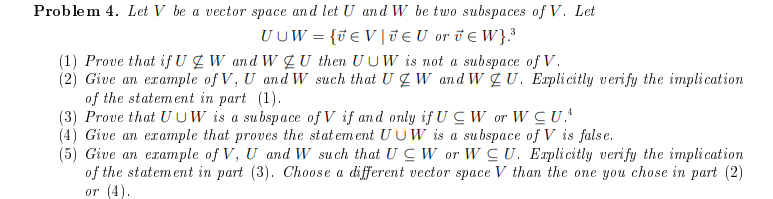1 Problem 4. Let V be a vector space and let U and W be two subspaces of V. Let (1) Prove that ifU W andWgU then UUW is not a subspace of V (2) Give an example of V, U and W such that U W andWgU. Explicitly verify the implication of the statement in part1). (3) Proue that...

• #### Let (X, d) be a metric space, and let ACX be a subset (a) (3 pts) Let x E X. Write the definition of d(x, A) (b) (7 pts) Assume A is closed. Prove that d(x,A-0 if and only if x E A. Let (X, d) b...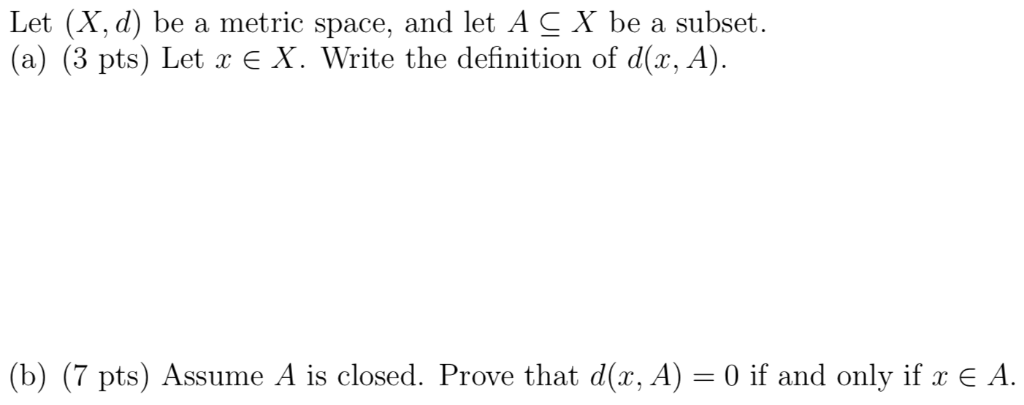Let (X, d) be a metric space, and let ACX be a subset (a) (3 pts) Let x E X. Write the definition of d(x, A) (b) (7 pts) Assume A is closed. Prove that d(x,A-0 if and only if x E A. Let (X, d) be a metric space, and let ACX be a subset (a) (3 pts) Let...

• #### Let X and Y be topological spaces, and let X × y be equipped with the product topology. Let yo E Y be fixed. Define the map f XXx Y by f(x) (x, yo) Prove that f is continuous, Let X and Y be top...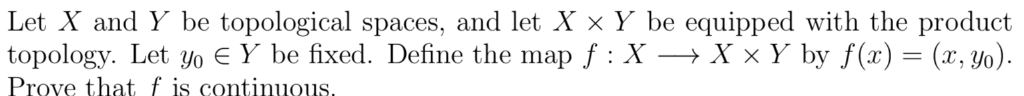Let X and Y be topological spaces, and let X × y be equipped with the product topology. Let yo E Y be fixed. Define the map f XXx Y by f(x) (x, yo) Prove that f is continuous, Let X and Y be topological spaces, and let X × y be equipped with the product topology. Let yo E...

• #### 12. (True/False) (a) Let AE Rm*n . Then R(A) (b) Let AERm*n. Then N(A) is isomorphic to N(AT) (c) We define < A. B > = Tr (BTA ) where A, B E Rnxn . is isomorphic to R(A Then 〈 . , . 〉 is an...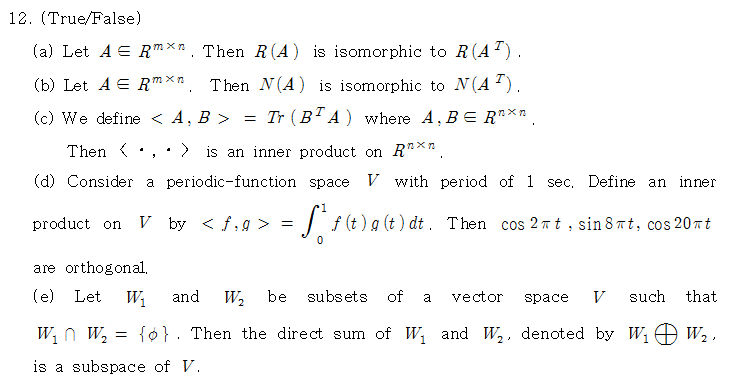12. (True/False) (a) Let AE Rm*n . Then R(A) (b) Let AERm*n. Then N(A) is isomorphic to N(AT) (c) We define < A. B > = Tr (BTA ) where A, B E Rnxn . is isomorphic to R(A Then 〈 . , . 〉 is an inner product on Rmxn. (d) Consider a periodic-function space V with period of...

• #### 22. Prove: if a, b, and c are odd, and a | b - c and a bc, then a | b and a c 22. Prove: if a, b, and c are odd, and a | b - c and a bc, then a | b and a c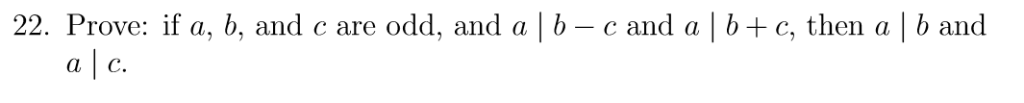22. Prove: if a, b, and c are odd, and a | b - c and a bc, then a | b and a c 22. Prove: if a, b, and c are odd, and a | b - c and a bc, then a | b and a c

• #### 13. Prove that for all integers b, if b is odd then b is odd 13. Prove that for all integers b, if b is odd then b is odd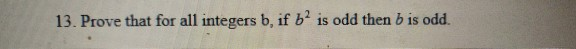13. Prove that for all integers b, if b is odd then b is odd 13. Prove that for all integers b, if b is odd then b is odd

• #### prove thsh f(x,y) then n exists 3, 、 2 2. prove thsh f(x,y) then n exists 3, 、 2 2.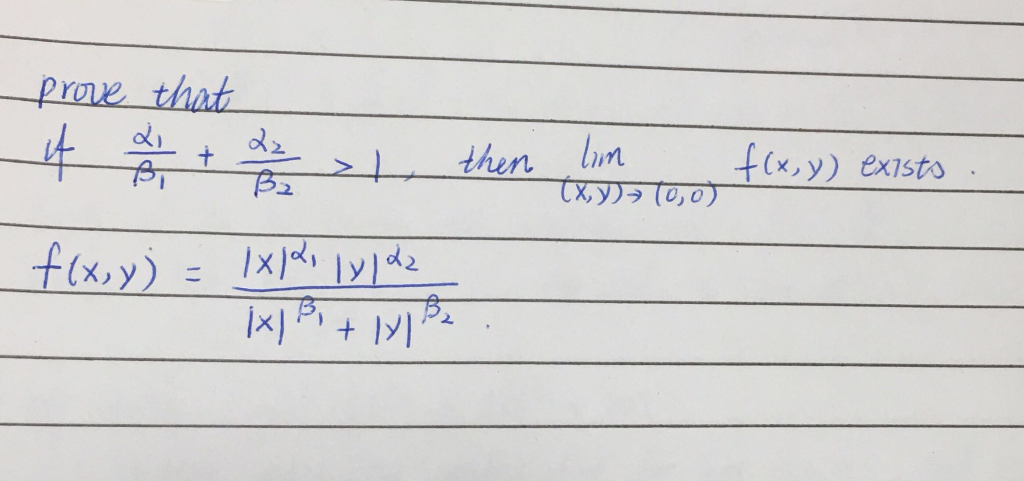prove thsh f(x,y) then n exists 3, 、 2 2. prove thsh f(x,y) then n exists 3, 、 2 2.

• #### z 또 Q then re Q Exercise 10. Prove or disprove: If z 또 Q then re Q Exercise 10. Prove or disprove: If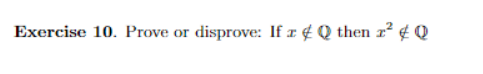z 또 Q then re Q Exercise 10. Prove or disprove: If z 또 Q then re Q Exercise 10. Prove or disprove: If

• #### a. Prove: If A and B are independent, then so are A and B. b. Prove: If A and B are independent, then so are and . c. G...

a. Prove: If A and B are independent, then so are A and B. b. Prove: If A and B are independent, then so are and . c. Give an example of events A, B, and C such that but We were unable to transcribe this imageWe were unable to transcribe this imageP(An Bn C) = P(A)P(B)P(C), P(AnBn C) P(A)P(B)P(C)...

• #### Let f be defined on an open interval I containing a point a (1) Prove that if f is differentiable on I and f"(a) exists, then lim h-+0 (a 2 h2 (2) Prove that if f is continuous at a and there...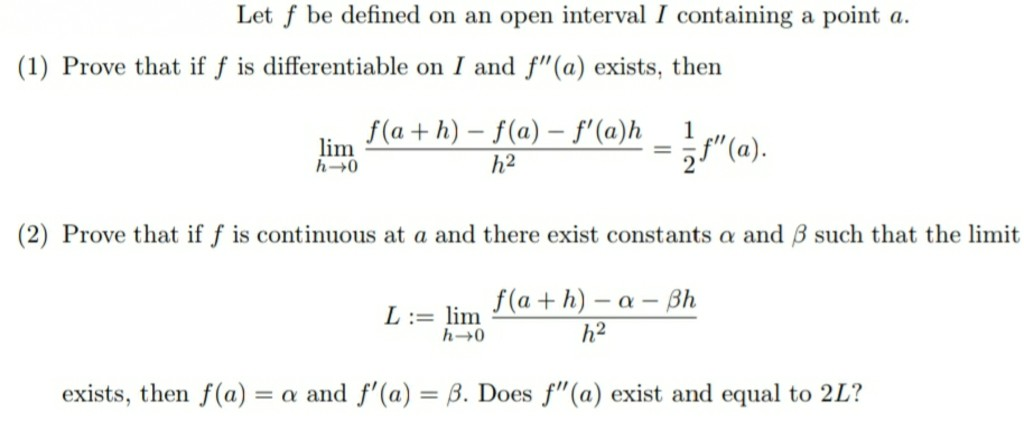Let f be defined on an open interval I containing a point a (1) Prove that if f is differentiable on I and f"(a) exists, then lim h-+0 (a 2 h2 (2) Prove that if f is continuous at a and there exist constants α and β such that the limit L := lim h2 exists, then f(a)-α and f'(a)-β....

• #### 3. Let X be a random variable and denote by Mx(t) its MGF. Prove that, for any t > 0, we have 3. Let X be a random variable and denote by Mx(t) its MGF. Prove that, for any t > 0, we have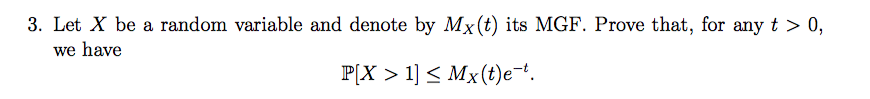3. Let X be a random variable and denote by Mx(t) its MGF. Prove that, for any t > 0, we have 3. Let X be a random variable and denote by Mx(t) its MGF. Prove that, for any t > 0, we have

• #### (1) Let (X,d) be a metric space and A, B CX be closed. Prove that A\B and B\A are separated (1) Let (X,d) be a metric space and A, B CX be closed. Prove that A\B and B\A are separated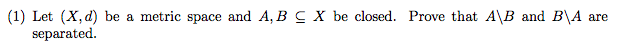(1) Let (X,d) be a metric space and A, B CX be closed. Prove that A\B and B\A are separated (1) Let (X,d) be a metric space and A, B CX be closed. Prove that A\B and B\A are separated

• #### (c) Let f :la,b- R be an integrable function. Prove that lim . (Your argument should include why faf makes sense for a < x < b.) (c) Let f :la,b- R be an integrable function. Prove tha...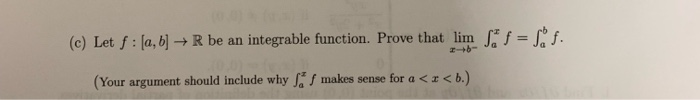(c) Let f :la,b- R be an integrable function. Prove that lim . (Your argument should include why faf makes sense for a < x < b.) (c) Let f :la,b- R be an integrable function. Prove that lim . (Your argument should include why faf makes sense for a

Free Homework App